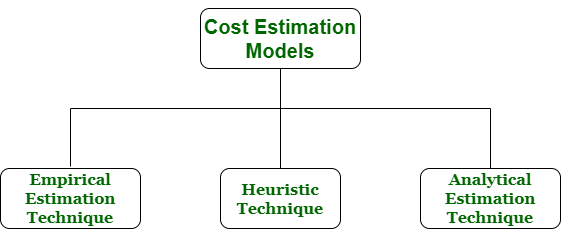# Cost Estimation Models in Software Engineering

Cost estimation simply means a technique that is used to find out the cost estimates. The cost estimate is the financial spend that is done on the efforts to develop and test software in Software Engineering. Cost estimation models are some mathematical algorithms or parametric equations that are used to estimate the cost of a product or a project.

Various techniques or models are available for cost estimation, also known as Cost Estimation Models as shown below :1. Empirical Estimation Technique –
Empirical estimation is a technique or model in which empirically derived formulas are used for predicting the data that are a required and essential part of the software project planning step. These techniques are usually based on the data that is collected previously from a project and also based on some guesses, prior experience with the development of similar types of projects, and assumptions. It uses the size of the software to estimate the effort.

In this technique, an educated guess of project parameters is made. Hence, these models are based on common sense. However, as there are many activities involved in empirical estimation techniques, this technique is formalized. For example Delphi technique and Expert Judgement technique.

2. Heuristic Technique –
Heuristic word is derived from a Greek word that means “to discover”. The heuristic technique is a technique or model that is used for solving problems, learning, or discovery in the practical methods which are used for achieving immediate goals. These techniques are flexible and simple for taking quick decisions through shortcuts and good enough calculations, most probably when working with complex data. But the decisions that are made using this technique are necessary to be optimal.

In this technique, the relationship among different project parameters is expressed using mathematical equations. The popular heuristic technique is given by Constructive Cost Model (COCOMO). This technique is also used to increase or speed up the analysis and investment decisions.

3. Analytical Estimation Technique –
Analytical estimation is a type of technique that is used to measure work. In this technique, firstly the task is divided or broken down into its basic component operations or elements for analyzing. Second, if the standard time is available from some other source, then these sources are applied to each element or component of work.

Third, if there is no such time available, then the work is estimated based on the experience of the work. In this technique, results are derived by making certain basic assumptions about the project. Hence, the analytical estimation technique has some scientific basis. Halstead’s software science is based on an analytical estimation model.

Attention reader! Don’t stop learning now. Get hold of all the important CS Theory concepts for SDE interviews with the CS Theory Course at a student-friendly price and become industry ready.

My Personal Notes arrow_drop_upCheck out this Author's contributed articles.

If you like GeeksforGeeks and would like to contribute, you can also write an article using contribute.geeksforgeeks.org or mail your article to contribute@geeksforgeeks.org. See your article appearing on the GeeksforGeeks main page and help other Geeks.

Please Improve this article if you find anything incorrect by clicking on the "Improve Article" button below.

Article Tags :

Be the First to upvote.

Please write to us at contribute@geeksforgeeks.org to report any issue with the above content.